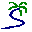# Model entry

Population dynamics: single species: chaotic - Model catalogue - Simulistics.com

 Search Simulistics Model catalogue Listed by keyword Listed by ID Listed by title Listed by date added

# Population dynamics: single species: chaotic

Model : chaos
Simile version : 3.1+
Keywords : Population dynamics ; Single-species population dynamics ; Chaotic behaviour ;

## Description

This version differs from the standard population model in two respects:

1. the growth rate depends on the current population by a quadratic relation. This will cause the population to approach the point of zero growth; and
2. we consider time as being discrete rather than continuous. Rather than using a very small time increment between model states it is set equal to the time unit.

The behaviour of this model approximates that of a population where new individuals arrive all at once at a certain time of year, and most deaths also occur within a short period each year.

X(t+1) = g X(t) (1 - X(t)/100)
where:
X(t) = population size (number of individuals, or number of individuals per unit area) at time t g = growth rate (number of new individuals for each existing one with no resource constraints)

## Files

### Model file

Click on the icon to download the model file. (You will need Simile to examine and run the model. A free evaluation version is available from the products page.)

Some browsers may attempt to display the model file, rather than open it in Simile; in this case, use the browser back button to return to this page, and use the context menu (invoked by right-clicking on the link) to save the target file to disk.chaos.sml

## Equations

```Compartment   level
Initial value = rand_const(50,50.000001)
Rate of change =  + change

Flow   change
change = gain*level*(1-level/100)
```This graph shows the results of four consecutive runs with gain set to 1.4, 1.9, 2.3 and 2.7 respectively. On the first two runs the level approaches equilibrium; on the third it oscillates around the equilibrium, and on the final run it shows chaotic behaviour. Other behaviours such as period doubling will be shown for other values of gain.This graph illustrates the unpredictability of chaotic behaviour. The model includes a very small random variation in the initial value of level, but the values for three consecutive runs, all with gain set to 2.7, diverge after about 60 time units. Until this time, the plot for the last run (green) exactly overwrites the other two.